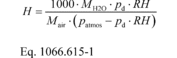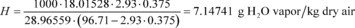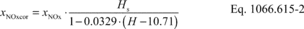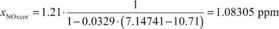# Title 40

## SECTION 1066.615

### 1066.615 NOX intake-air humidity correction.

§ 1066.615 NOX intake-air humidity correction.

You may correct NOX emissions for intake-air humidity as described in this section if the standard-setting part allows it. See § 1066.605(c) for the proper sequence for applying the NOX intake-air humidity correction.

(a) For vehicles at or below 14,000 pounds GVWR, apply a correction for vehicles with reciprocating engines operating over specific test cycles as follows:

(1) Calculate a humidity correction using a time-weighted mean value for ambient humidity over the test interval. Calculate absolute ambient humidity, H, using the following equation:Where: MH2O = molar mass of H2O. pd = saturated vapor pressure at the ambient dry bulb temperature. RH = relative humidity of ambient air Mair = molar mass of air. patmos = atmospheric pressure. Example: MH2O = 18.01528 g/mol pd = 2.93 kPa RH = 37.5% = 0.375 Mair = 28.96559 g/mol patmos = 96.71 kPa(2) Use the following equation to correct measured concentrations to a reference condition of 10.71 grams H2O vapor per kilogram of dry air for the FTP, US06, LA-92, SC03, and HFET test cycles:Where: χNOx = measured NOX emission concentration in the sample, after dry-to-wet and background corrections. Hs = humidity scale. Set = 1 for FTP, US06, LA-92, and HFET test cycles. Set = 0.8825 for the SC03 test cycle. H = ambient humidity, as determined in paragraph (a)(1) of this section. Example: H = 7.14741 g H2O vapor/kg dry air time weighted over the FTP test cycle χNOx = 1.21 ppm(b) For vehicles above 14,000 pounds GVWR, apply correction factors as described in 40 CFR 1065.670.

[80 FR 9121, Feb. 19, 2015, as amended at 81 FR 74207, Oct. 25, 2016]